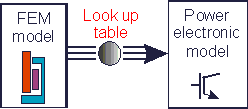• Introduction
• What is in this manual
• What is Caspoc
• User interface
• Introduction
• Starting
• Simulation
• Editing
• Viewing and printing
• Getting Started
• Basic editing
• Simulation in the time domain
• Basic User Interface Topics
• Editing
• Simulation
• Viewing
• Library
• Reports
• Project management
• Circuit and Block Diagram Components
• Introduction
• Cscript and user defined functions
• Component parameters
• Modeling Topics
• Introduction
• Power Electronics
• Semiconductors
• Electrical Machines
• Electrical drives
• Power Systems
• Mechanical Systems
• Thermal Systems
• Magnetic Circuits
• Green Energy
• Coupling to FEM
• Experimenter
• Analog hardware description language
• Embedded C code Export
• Coupling to Spice
• Small Signal Analysis
• Matlab coupling
• Tips and tricks
• Appendices

## FEM Coupling look up tables.

Flux linkage or co-energy tables from static FEM analysis can be created that are used in the system simulation using look up tables.Look up tables exchange is a method where the first tool is used to design or analyze a component and use the resulting look up tables from that analysis in a model in the second tool. Depending on the results from the second tool, the user can make modifications in the model in the first tool and repeat the analysis in the first and in the second tool.Example Permanent Magnet Synchronous Machine

To study the behavior of Permanent Magnet Synchronous Machines(PMSM), the back-emf and torque produced by the machine contain a number of higher harmonics. Instead of using the basic-harmonic model, results from FEM are used to enhance the PMSM model. In that case the back-emf and torque waveform are calculated in FEM as a function of the rotor position.

The snapshot from the machine model below shows how the lookup blocks are connected to the back-emf controlled voltage sources.In this example a one-dimensional lookup table is used to model the rotor position dependent back-emf constant. This constant is multiplied by the number of poles pairs and with the rotor angular speed.